### Physically-Based Fluid Modeling using Smoothed Particle Hydrodynamics

Chapter 3: Implementation

### Particle Systems and Fluid Dynamics

This new method of modeling fluid has roots in two previously unrelated areas: particle systems from computer graphics, and Smoothed Particle Hydrodynamics (SPH) from computational fluid dynamics (CFD). This chapter presents an overview of each of these areas.

#### 2.1. Introduction to Particle Systems

A particle is a point in three-dimensional (3D) space which has attributes such as mass and velocity. A particle system is a collection of particles which are dynamically changing over time as a result of external forces or some stochastic process. Particle systems were introduced into computer graphics in 1983 by Reeves as a method of modeling "fuzzy" objects such as fire, smoke and grass (Reeves, 1983). As mentioned earlier, particle systems have also been used for modeling waterfalls, ship wakes and breaking waves (Peachy, 1986; Fournier, 1986; Goss, 1990; Sims, 1990). These systems consist of independent particles which do not interact with each other. When particles interact it is possible to model phenomena such as flocking (Reynolds, 1987) and viscous fluids (Miller, 1989; Terzopoulos, 1989; Tonnesen, 1991). These systems are referred to as spatially coupled particle systems (Tonnesen, 1992), since the interaction between two particles is a function of their spatial relationship. The work presented here involves a coupled particle system and so this section will focus on this type of system.

#### 2.1.1. Particle Attributes

The attributes of particles can vary widely depending on the object(s) being modeled. Common characteristics involve the following:
• position
• mass
• velocity
• acceleration
• size
• color
• age
• temperature
Each particle must have at least a position and velocity. Particles can also have orientation which assigns each particle a local coordinate system (Szeliski and Tonnesen, 1992), causing particles to arrange themselves into surfaces instead of solids.

Particles are moved by applying forces and calculating the acceleration. Other attributes can change over time as well, which can enhance the representation of complex, dynamic objects.

#### 2.1.2. Particle Dynamics

The most common particle is a Newtonian particle whose motion is governed by Newton's laws of motion (Witkin, 1993). The position of a particle can be written as a function of time: x(t). The velocity is defined as the rate of change of the position over time: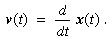Acceleration is then the rate of change of velocity over time: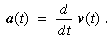Accelerations are calculated from forces applied to the particles.

The animation of a particle system using Newtonian dynamics can be summarized as follows:

```    for each time step
calculate forces on all particles
for each particle
calculate acceleration:integrate equations of motion: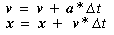```
The variable f represents the force vector, and m is the mass. The integration shown above is known as Euler's method. It is the simplest and most common method, but is unstable, inaccurate and inefficient (Witkin and Baraff, 1993). Other higher order methods such as Runge-Kutta and midpoint are more accurate, and more stable. The system presented here uses the leap-frog method which will be discussed in detail in Section 3.3.

Forces acting on particle systems commonly include gravity and damping: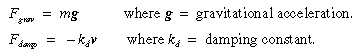Coupled particle systems are implemented by introducing binary forces which act on pairs of particles. A simple example is that of a spring: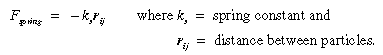A spring applies to a fixed connection between two particles. In contrast, forces can be defined which act over all particle pairs. In this situation particle interaction increases as two particles move closer together, and decrease as they move further apart. This idea stems from molecular dynamics, where long-range attraction forces and short-range repulsive forces determine the movement of molecules. This is commonly modeled using the Lennard-Jones potential energy function (Sprackling, 1985) which takes the form:The first term models repulsion, the second attraction. Most literature reports using n = 12, m = 6, or smaller (Miller, 1989; Tonnesen, 1991). A and B vary according to the specific problem being solved.

The molecular dynamics approach has been useful in modeling fluid behavior (Miller, 1989; Terzopoulos, 1989; Tonnesen, 1991). A disadvantage to this type of force is that the calculation is O(N�), where N is the number of particles. This can be alleviated somewhat by limiting interactions to nearest neighbors, and through the use of creative data structures.

#### 2.1.3. Collision Detection and Response

Handling collisions between particles and obstacles in their environment is not a simple task. First a system must detect the collision, then must respond in such a way that the movement of the particle is still believable. Although there are many methods that have been used in computer animation, only a few of the more popular methods are described here. The literature on collision handling is extensive; Moore and Wilhelms (Moore and Wilhelms, 1988) offers a comprehensive discussion of several methods.

A collision is detected by checking the position of a particle against the plane made by the polygon in question. Witkin (1993) states:

Although general collision detection is hard, particle/plane collision detection is trivial. If P is a point on the plane, and N is a normal, pointing inside (i.e. on the legal side of the barrier,) then we need only test the sign of (X - P)*N to detect a collision of point X with the plane. A value greater than zero means it's inside, less than zero means it's outside (where it isn't allowed to be) and zero means it's in contact. (p. C11)
A simple collision response involves modifying the normal component of the velocity vector. For a perfectly elastic collision (e.g. a golf ball) the normal component is simply negated. For softer collisions (e.g. jello) it can be multiplied by a constant r between 0 and 1. With r set to 0, the particle will not bounce at all. A contact force can be used to prevent a particle resting on the surface from being pushed into the surface: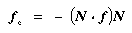which essentially cancels the normal component of the incoming force.

The idea of surface forces can be extended to the use of repulsive forces for preventing a particle from ever coming into contact with the obstacle. In essence a "force field" is set up around the obstacle by using a function which exponentially increases as the distance between the particle and the obstacle decreases (Terzopoulos et al., 1987; Monaghan, 1994).

Inserting a spring at the point of contact is another common collision response. This method is intuitive but computationally expensive. Stiffer springs require stiff equations and therefore require very small time steps (Moore and Wilhelms, 1988).

#### 2.1.4. Particle Rendering

Once the particle positions have been determined, the last step is to render the scene. There are several methods which can be classified into two categories: rendering individual particles, or rendering the surface of the object the particles are representing. Rendering individual particles works well for modeling waterfalls or spray, and is usually faster but can only give a general idea about liquid or other continuous materials. A surface rendering will give a more accurate description of the object if it is made of some cohesive substance (i.e. slime vs. fireworks), but is generally much slower, especially with a system that is changing over time.

Individual particles can be rendered quickly and easily as point light sources or streaks of light. They can also be rendered using primitives such as spheres or cubes which can be drawn using either solid or wire frame models.

Surface rendering is a bit more complicated. Since a particle system does not specifically describe a surface, one must be computed. This can be done using implicit surfaces. Implicit surfaces are a class of curved surfaces which are defined as being the solution to some equation:To generate a surface around a single point you can define a density field for that point. The field is generated using a function D whose value decreases exponentially as the distance from the point increases. An implicit surface would then be defined as the set of points where the density function is equal to some threshold T:This method was introduced by Jim Blinn in 1982 who coined the term "blobbies" in reference to the resulting shape of the objects (Blinn, 1982). It has been used to model many types of deformable and soft objects (Wyvill et al., 1986a) including particle-based fluid (Miller, 1989; Tonnesen, 1991). The implicit surface can be rendered directly using ray-tracing or can be polygonized using well-known polygonization algorithms (Wyvill, 1986b; Lorensen and Cline, 1987; Bloomenthal, 1988).

The algorithm presented here renders the liquid model as a polygonal approximation of the surface defined by the particle system, with the option for individual particle rendering. The surface generation and polygonization are discussed in Section 3.5.

#### 2.2. Introduction to Fluid Dynamics

It is generally understood that there are three phases of matter: solid, liquid and gas. A solid is rigid, with definite shape, where a liquid will take the shape of its container while maintaining a constant volume, and a gas will completely fill its container, taking the shape of the container's boundaries. At a microscopic level, the molecules of a substance are constantly vibrating about some mean position. The amount of this vibration describes the matter's internal energy, and therefore determines its state. In a solid the energy is low enough (i.e. the molecular vibration is small enough) that the molecules maintain their position relative to each other, and so maintain a regular arrangement or structure which gives a solid its rigidity. In a liquid there is enough energy for the molecules to break apart and move past each other (slowly), but the vibrations are not strong enough to completely get away from the attractive forces holding the molecules together. In a gas state the vibrations are large enough that the molecules break away from each other entirely and can move about freely. In general a "fluid" refers to a substance in either the liquid or gas state.

The mean pressure (P) on an area of fluid is defined as the ratio of the normal force acting on the area to the area. The pressure at a single point in the fluid is the limit that the mean pressure approaches as the area approaches zero (John, 1988). This can be demonstrated by looking at the force acting on a cubical element of fluid, as shown in Figure 1. Pressure can be exerted on an element by surrounding fluid elements or by the atmosphere if a surface is exposed to air.Figure 1. Pressure on a small volume of fluid.

The mean density (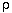) of a volume of fluid is defined as the mass within a volume V divided by that volume. The density at a point is the limit as the volume approaches zero: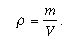Density is related to the temperature of the fluid and the pressure exerted on the fluid elements: it will increase as pressure increases or as temperature decreases. The reaction to a change in pressure or temperature is different for a liquid than for a gas. When surrounding pressure is increased, a volume of gas will decrease substantially, causing a large change in density. When the same pressure change is applied to a similar volume of liquid, the volume will only decrease by a small amount, causing very little change in the density. The difference is due to the compressibility of the substance. A gas is much more compressible than a liquid, which means that its density can vary widely with pressure changes. The density fluctuations for a liquid are so small that a liquid is generally considered incompressible. The term "compressible fluid" will be used to refer to a gas, and "incompressible fluid" will refer to a liquid.

Fluid dynamics is the study of fluid motion in response to forces such as gravity and pressure. The equations of motion for a compressible fluid can be written in the form:Equation 2.1 is referred to as the continuity equation. It describes the conservation of mass as the fluid evolves over time. Equation 2.2 is the momentum equation, which describes the conservation of momentum (mass multiplied by velocity), and equation 2.3 is the energy equation, describing the conservation of energy (John and Haberman, 1988; Sprackling, 1985).

In addition to these equations an equation of state must be used in order to fully describe the behavior of fluid. This equation defines a functional relationship between temperature, density and pressure (which varies according to the material being simulated). The form used for an ideal gas, sufficient to describe many compressible fluids, is: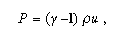where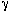is the ratio of specific heats, a parameter which depends on the particular gas being simulated.

A computational model of fluid involves representing the fluid as a set of small finite-sized elements, each representing a small volume of the fluid. The equations of motion can then be applied to the elements to simulate the behavior of the fluid over time. Tracking behavior and attributes as fluid flows past elements fixed in space is the Eulerian approach to describing fluid motion. Tracking the behavior of fluid elements as they move with the flow is the Lagrangian approach. Traditional CFD methods such as the Finite Element Method (FEM) and Finite Differences (FD) use the Eulerian approach. The work presented here is based on a Lagrangian CFD method known as Smoothed Particle Hydrodynamics (SPH). A brief comparison of the three methods, and a detailed description of SPH follows.

#### 2.2.1. Computational Fluid Dynamics

The Finite Element Method and Finite Differences are often called "grid-based" methods, referring to the grid-like arrangement of the fluid elements being simulated. The fluid elements are stored in an array of cells, each representing one fluid element. The flow of fluid from one cell to the next is computed, and information such as pressure or temperature is updated for each cell. The Finite Element Method differs from Finite Differences in the grid structure. An FD grid is always a logically cubical array, sometimes termed a "structured grid". Its output consists of the physical parameters for each cell. An FEM grid is typically unstructured, with arbitrary and sometimes dynamic cell configurations. Its output is the same as FD, plus the vertices of the cell. The FEM method is more often used in modeling fluid flow around objects, since an unstructured grid makes it easier to examine the flow accurately near curved surfaces since the mesh can be "deformed" to create arbitrary surfaces.

Smoothed Particle Hydrodynamics also represents fluid as a collection of elements with local fluid characteristics. But, instead of calculating flow from one element to the next, the actual movement of these elements in response to external forces is computed. Each one of these elements, termed a "particle", has a mass, position and velocity and is influenced by forces such as gravity, similar to the elements of the particle systems described earlier. However, an SPH particle also has local fluid characteristics such as density and temperature, and responds to pressure forces from surrounding particles.

#### 2.2.2. Smoothed Particle Hydrodynamics

The SPH method was introduced in 1977 by Lucy (Lucy, 1977) and Gingold and Monaghan (Gingold and Monaghan, 1977) as a new method of solving the equations of motion of a compressible fluid. The equations used for the current work are from the adaptation of SPH for incompressible flow described by Monaghan in (Monaghan, 1992; 1994).

The idea behind SPH is the determination of characteristics of fluid by interpolating from a set of non-ordered points: the particles. The interpolation is performed using a smoothing kernel W which is a weighted sum over particles within an area defined by a smoothing length h. As an example, the smoothed estimate of the density at particle i can be found using:There are a number of possible choices for W , however the most advantageous (Monaghan, 1992) is: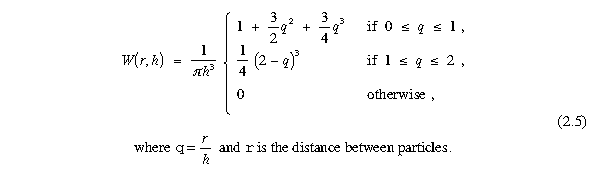Note that as q approaches 2 the value of W goes to 0 so that particles do not interact outside of two smoothing lengths. This fact improves the efficiency of the force calculations. Computing interactions among a restricted set of particles instead of across all particles can greatly reduce the number of computations per time step. Other methods of improving performance are discussed at the end of this section.

Computing the gradient of an interpolated value is done using the gradient of the smoothing kernel, giving a smoothed estimate of the gradient of the variable. The pressure gradient needed to compute the force on a particle is estimated using:This is the manner in which the SPH versions of the equation of motion are derived: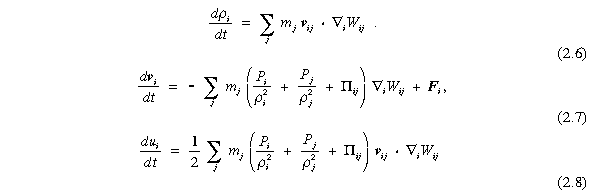The termis an artificial viscosity added to handle shocks. There are several variations of this expression. The version implemented for this research is from (Monaghan, 1992):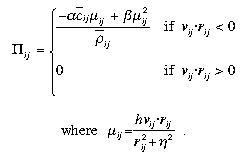Hereis the mean density for particles i and j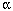and � are viscous constants (usually set to 1 and 2 respectively),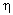is a constant used to prevent numerical divergences, and c is the mean sound speed.

The speed of sound for a particle i is:which represents the speed at which sound travels through the fluid element represented by the particle.

Using these equations the particle positions and attributes are advanced using standard numerical integration methods such as leap-frog or predictor-corrector, and a variable time step. The size of the time step is controlled by the "Courant condition" which basically says that the time step should be smaller than the length of time it takes sound to travel over a specific distance. In SPH this distance is the smoothing length h. Section 3.3 describes the time step algorithm used in this research.

When updating the density, either equation 2.4 or 2.6 can be used. Most SPH calculations use equation 2.4 but equation 2.6 is more appropriate for incompressible flow (Monaghan, 1992; Monaghan 1994). The reasoning for this is discussed in section 2.2.3.

To put all of these equations in perspective, it may be helpful to summarize the steps a typical SPH simulation goes through. Particles are initialized in a configuration suitable to the problem being solved (e.g. evolution of matter orbiting the center of a galaxy). They are given an initial position, velocity, mass and energy and the system is evolved in a manner similar to the following pseudo code:

```    Update particle densities
Update particle pressures
while (time < end_of_time)
for all particles
Calculate acceleration due to pressure gradient (equation 2.7)
Calculate rate of change of thermal energy (equation 2.8)

for all particles
Update position
Update velocity
Update thermal energy

Update particle densities
Update particle pressures
Calculate new time step
time += new_timestep```
Simulations in three-dimensions are usually run with 100 to 1000 particles. (Monaghan, 1992; 1994; Hernquist, 1989). The large number of particles combined with the inter-particle interaction algorithm results in a large amount of computations during every time step. This bottleneck can be alleviated by vectorizing or parallelizing the computations and/or using data structures which lend themselves to efficient nearest-neighbor traversal. Since particles only interact within two smoothing lengths the range of force calculations can be limited to "nearest neighbors." Algorithms based on linked lists or tree structures are commonly implemented for this type of computation (Theuns and Rathsack, 1993; Rhoades, 1992; Hernquist and Katz, 1989). A linked list implementation partitions particles into cubical cells which are one to two smoothing lengths wide. Interactions are then limited to particles in neighboring cells. Alternatively, particles can be stored in a hierarchical tree structure which encodes spatial relationships between particles.

#### 2.2.3. Adaptation For Incompressible Flow

Studying motion of an incompressible fluid (such as water) involves either solving the equations for incompressible flow (the Navier Stokes equations) or using the compressible equations (Euler's equations described earlier) to simulate a very stiff (nearly incompressible) gas. The computational complexity of the Navier-Stokes equations makes them difficult to solve efficiently, therefore Euler's equations are the preferred method for simulating incompressible flow.

In order to make a compressible fluid act as an incompressible fluid the pressure needs to be increased to a point where the density becomes so high that it is difficult to compress it any further. At this point small changes in density will result in very large changes of pressure, and conversely it will take a large amount of pressure to change the density by a small amount. The problem with such high pressures is that the Courant condition would now dictate extremely small time steps in the numerical integration.

Monaghan (Monaghan, 1994) uses this approach to adapt the SPH equations for incompressible flow. However, instead of approximating the real (incompressible) fluid with an exactly incompressible artificial fluid, he uses an artificial fluid which is more compressible than the real fluid. In other words, the simulated fluid is nearly incompressible, not entirely incompressible. This allows the time step size to be a little more reasonable, while still approximating a very stiff gas.

Incompressible SPH equations differ from traditional SPH equations in the density calculation, the velocity calculation and the equation of state. The standard density calculation (equation 2.4) is problematic because the density of liquid drops to zero at flow boundaries. If a smoothed estimate of that density is calculated using equation 2.4 the boundary particles will have a low density resulting in erroneous pressures from the equation of state. To alleviate this the particles are initialized with a given density and a rate of change for density is calculated based on the particles' relative velocity (equation 2.6)

The velocity of each particle is also adjusted to prevent particle interpenetrations and mixing. The XSPH variant (Monaghan, 1994; 1989) is used to coax particles to move with a velocity similar to the average velocity in its neighborhood which is said to be useful for high speed flow. It also helps keep particles of an incompressible flow orderly in the absence of viscosity. The variant is calculated using:This variant is added to the velocity when advancing the particle position, but does not affect the velocity used for other SPH calculations.

Monaghan also uses a different equation of state, similar to that defined for water: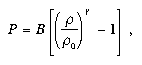This equation produces large changes in pressure for small changes in density, but if the system is in equilibrium (0 ) then the pressure becomes zero, which allows for larger time steps during integration. (Note: During implementation of the SPH code presented here equation 2.11 was found to be incorrect. See section 3.3)

Particle initialization and time stepping are the same as the original SPH (excepting the initial density mentioned earlier). When running a simulation Monaghan also uses damping to allow the system to settle into equilibrium before introducing perturbations (e.g. gravity or boundary movement) which initiate the motion to be studied.